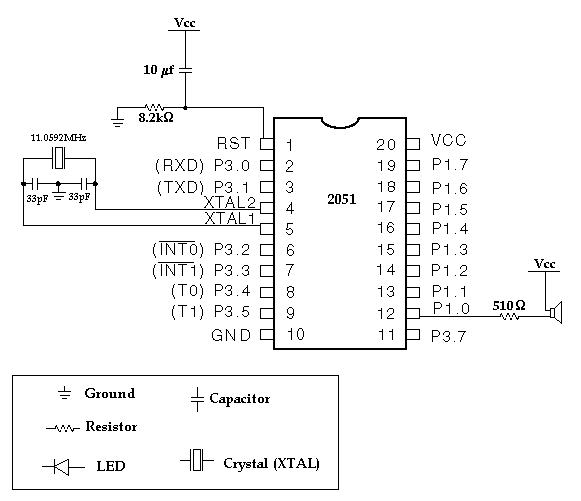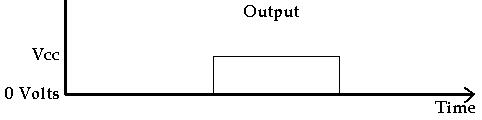� Experiment � Calculator/Converters � Radio � Newsletter � Associations and Societies � Component ManufacturersElectronics Symentics A B C D E F G H I J K L M N O P Q R S T U V W X Y Z

Microcontroller Intermediate Kit - Making Sound With The 2051

 The first step is to build the circuit. This design is intended for use with an Atmel 2051 programmable microcontroller (a 20 pin version of the 8051 microcontroller). An 8051 or 8052 can be substituted for the 2051 if the connections are moved to the appropriate pins on those chips. Vcc = 5V and Gnd = 0VThe basic process of compiling a program written in assembly language and then programming the resulting file into the microcontroller was covered in the first microcontroller tutorial.  This project uses the same code as the first program. To start, we just make a minor change to the original assembly language program, ledtest.asm.  Replace the line at the bottom that says ACALL DELAYHS with ACALL DELAYMS. This changes the delay from half a second to one millisecond.  Save it as a new file called sounds.asm. That change makes it so that rather than delaying a half a second, we are only delaying one millisecond. Compile the sounds.asm file, load sounds.hex into the 2051 and put the chip back in the circuit. Connect the power. You should hear a tone coming from the speaker. If the LED was hooked up, it would be going on and off so fast that it would look like it was on all the time. But with the speaker, we can hear the voltage going on and off. You can only see the LED going on and off up to about 25 blinks per second. You can start to hear the speaker making noise at about 50 blinks per second. But instead of calling it blinks, we call it cycles. Right now the microcontroller is putting out about 500 cycles per second. This is also called the frequency. It is a frequency of 500 Hertz (where Hertz means cycles per second). If you want to make the frequency go down, you can add more delay. Try adding another millisecond delay by inserting another ACALL DELAYMS. This will make the frequency 250 Hertz. You can hear that the sound is lower in frequency. How to get exactly the frequency you want: To figure out how to get an exact frequency, you have to carefully look at how long it takes for the microcontroller to switch the output from 0 volts to 5 volts and back to 0 volts again. This is one cycle.Lets start by determining what frequency the sounds.asm program is creating. First lets look at the DELAYMS routine. We need to figure out how long this routine lasts. By just looking at the code, we can figure out that it goes through a loop 255 times. Each time through the loop it does 3 commands. The first two commands each take 12 clock cycles and the 3rd command (CJNE) takes 24 clock cycles. So each loop takes 48 clock cycles. To translate this into time, we need to look at the clock speed of the microcontroller. We are using an 11.0592 MHz crystal. This means the clock is running at a frequency of 11,059,200 cycles per second. (MHz is MegaHertz which is millon cycles per second). Each cycle takes 1/11,059,200 seconds = 0.00000009 seconds. So each loop takes 48 * 0.00000009 = 0.00000434 seconds. And 255 loops takes 255*0.00000434 = 0.001106771 seconds which is slightly longer than 1 millisecond (1 millisecond = 0.001 seconds). If we wanted to get closer to exactly 1 ms we could change the loop so that it only repeats 230 times rather than 255. So, the program makes the output go from 0 to 5 volts, then waits 1 ms, then goes from 5 volts to 0 volts, then waits 1 ms and that makes one cycle. So one cycle takes about 2 ms (This is called the period). To convert that to frequency, divide 1 cycle by 2 ms (1/0.002 = 500). Then you get 500 cycles per second. Or, to be exact, using the numbers above, one cycle takes 2.213542 ms for a frequency of 451.76 Hz. So, to get an exact frequency, you can start with the frequency and work backwards. Say you want to make 440 Hz, which is a musical  A note. To find the period, divide 1 by 440. This gives you the period equal to 0.002272727 seconds. Then divide this by 2 to find out how long each delay must be (there will be 2 delays per cycle). Each delay should be 0.001136364. Then find out how many microcontroller clock cycles this is by dividing by 0.00000009. This equals 12626 cycles (after dropping the decimal part). Using our loop that takes 48 cycles, this would be about 263 loops (12626 / 48 = 263). We can only go up to 255 loops so then we can either make our loop take more time, or add in an extra DELAY routine that adds in the extra 8 loops. The easiest solution is to make our loop longer. We can add in an extra 12 cycles per loop by putting in a NOP (no operation) command. Then each loop is 60 cycles and we need about 210 loops (12626 / 60 = 210). The resulting code is shown in sound440.asm. That will not be exactly 440 Hz because we had to round off in some places (you can't do 210.43 loops but 210 is close. To figure out exactly what frequency that we made, we can do the same as we did above with the DELAYMS routine. Each loop is 0.00000009 * 60 = 0.0000054 seconds. Each DELAYMS takes 210 * 0.0000054 = 0.001134 seconds. With 2 delays per cycle this is a period of 0.002268. In terms of frequency, 1/0.002268 = 440.9 cycles per second which is close to 440. Note: To really be exactly right on the frequency you are making, you need to include the time in each cycle for the other commands, CPL, ACALL and RET, and the commands in the DELAYMS loop, MOV and RET. These add an extra 96 clock cycles each time through. Since it takes 2 times through to make a cycle on the output, That is an extra 192 cycles. This equals 192 * 0.00000009 = 0.00001728 seconds. So the period is actually 0.002268 + 0.00001728 = 0.00228528 and the frequency is actually 437.6. So this extra time must be considered if you are trying to get a very precise frequency. Note: You are limited in how close you can get to an exact frequency by the microcontroller clock speed. The faster the clock is, the more accurate you can be. For example, with a 11.0592 MHz clock where each cycle is 0.00000009 seconds, the closest you can get to 440 Hz is 440.0788621 Hz. This is found by 1/440 = 0.002272727 seconds and 0.002272727 / 0.00000009 = 25253 cycles (must round to closest whole number because you can't have part of a cycle). Since the shortest commands take 12 clock cycles, then you won't be able to write a routine that takes exactly 25253 cycles. It has to be some multiple of 12. The closest multiple of 12 is 25248. Then 25248 * 0.00000009 = 0.00227232 seconds and 1 / 0.00227232 = 440.0788621 Hz. If you have a faster microcontroller clock speed you can be more accurate. For example, with a 24 MHz clock (The fastest you can use with a 2051 microcontroller) then you can get 440.0440044 Hz. Also, if you use a clock that gives you a different period you may be able to get exactly 440 Hz. For example, if you have a microcontroller clock that is 22,440,002.69 MHz then you can get much closer to 440 Hz, but you have to find a crystal that runs at that exact speed, and there probably is not one.